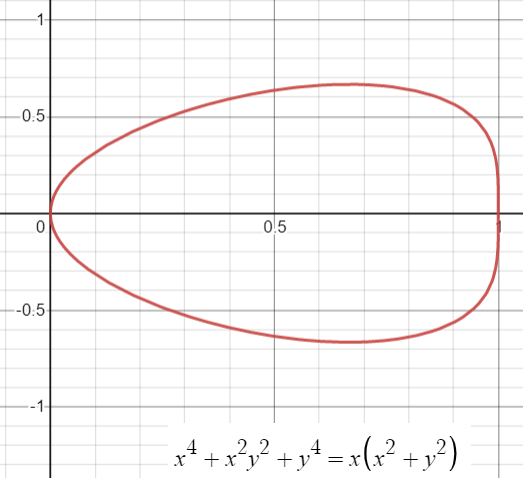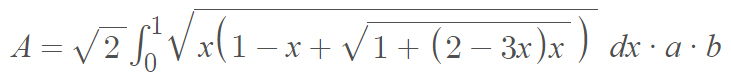# Bean CurveThe bean curve, named because it looks like a bean, is a quartic plane curve situated in the 1st and 4th quadrants with the origin at one end of the bean. It is defined by the implicit equation: x4 + x2y2 + y4 = x(x
2 + y2) .

There is one horizontal asymptote, at (2/3 a ± 2/3a) and two vertical asymptotes at (0, 0) and (a, 0).

## Area of the Bean Curve

The area of the bean curve is given by the integral :## Alternative Bean Curve

The curve defined above is the simplest type of bean-shaped curve. However, there is an alternative bean, described by the Cartesian equation (x2 + y2)2 = x3 + y3, shown below.This alternate curve can be described by the polar equation
r = a(sin3θ + cos3θ).

## Bean Curve History

The origins of the bean curve are somewhat of a mystery. One early mention of the curve was in Cundy and Rollett’s 1961 text Mathematical Models , where it had a one line entry as an “interesting quartic” with just the label “bean” and its Cartesian equation (p.72).

The first curve listed above is sometimes called the egg curve, presumably to differentiate it from the alternate bean-shaped curve. However, this can get a bit confusing as the alternate curve is sometimes called the “crooked egg curve” (even though it clearly looks like a lima bean!). None of this really matters, because the use of both curves seems to be mostly an academic pursuit, with very few (if any) real life applications.

## References

Bean curve graphed with Desmos.
 Knill, O. & Chi, A. (2003). Entry Curves. Retrieved December 31, 2021 from: https://abel.math.harvard.edu/~knill/sofia/data/curves.pdf
 Vaze, V. et al. (2005). Continuous space representations of human activity spaces. Arbeitsberichte Verkehrs- und Raumplanung.
 Cundy, H. & Rollett, A. (1951). Mathematical Models. Oxford University Press.

CITE THIS AS:
Stephanie Glen. "Bean Curve" From StatisticsHowTo.com: Elementary Statistics for the rest of us! https://www.statisticshowto.com/bean-curve/